# On solutions of functional equations with polynomial translations

### Full PDFcreative_2019_28_1_53_59

In this paper, we study polynomial functional equations of the form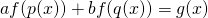,
where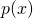,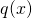are given polynomials and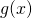is a given function. Theorems 21 and 22 contain sufficient conditions under which the functional equation has a solution of the special form. In Section 3 we present an algorithm of constructing polynomial solutions of the functional equations. Other non-polynomial solutions depend on solutions of the homogeneous equation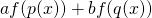=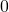. That case is analyzed in Section 4. Finally, we present a simple method of constructing examples with desirable properties.